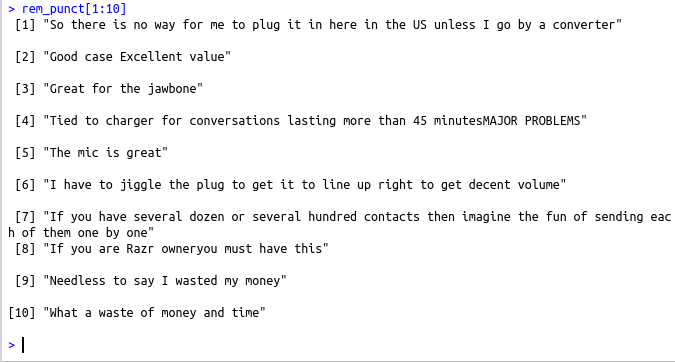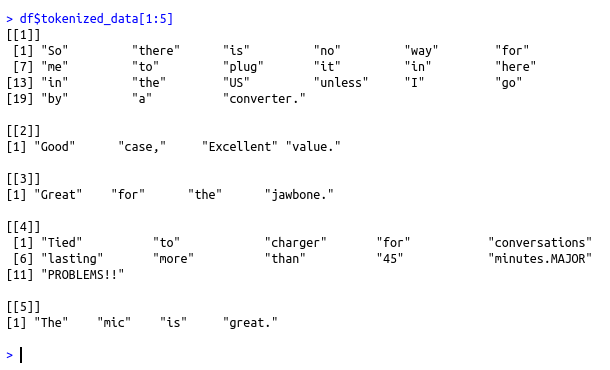• #5, First Floor, 4th Street Dr. Subbarayan Nagar Kodambakkam, Chennai-600 024 Landmark : Samiyar Madam
• pro@slogix.in
• +91- 81240 01111

#### Social List

##### How to Tokenize a given text and remove punctuation using the regular expression in R?
###### Description

To Tokenize a given text and remove punctuation using the regular expression in R

#### Functions Used

strsplit(data,” “) – To Tokenize the text by splitting it using space
gsub(“[[:punct:]]”,””,data) – To remove punctuation

###### Process

Transform the data set into a format as required(Skip this step if it is in proper format)

Tokenize the text by splitting the text using the space character

Find where the punctuation are present and replace it with an empty character

###### Sapmle Code

data data1=(strsplit(data\$text,”\n”))
data2=unlist(data1[])
data3=strsplit(data2,”\t”)
data4=unlist(data3)
i=0
j=0
k=0
text=c()
pol=c()
for (i in (1:length(data4)))
{
if(i%%2!=0)
{
j=j+1
text[j]=data4[i]
}else
{
k=k+1
pol[k]=data4[i]
}
}
df=data.frame(text=text,polarity=pol,stringsAsFactors = FALSE)
#Split the data with the space character to tokenize each word
v1=strsplit(df\$text,” “)
#Now add the tokenized data as a new column to the existing data frame
df\$tokenized_data df\$tokenized_data
#To remove the punctuation
rem_punct=gsub(“[[:punct:]]”,””,df\$text)
df\$text[1:10]
rem_punct[1:10]

###### Screenshots